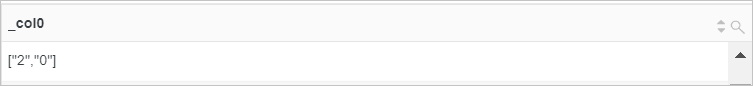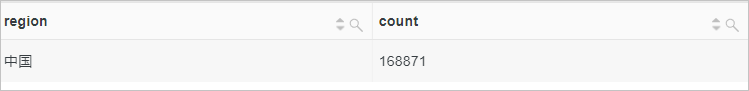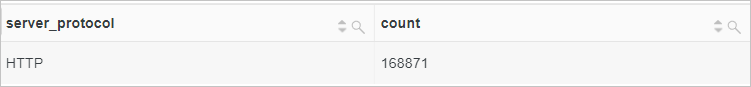This topic describes the syntax of regular expression functions. This topic also provides examples on how to use the functions.

The following table describes the regular expression functions that are supported by Log Service.

Notice If you want to use strings in analytic statements, you must enclose strings in single quotation marks (''). Strings that are not enclosed or enclosed in double quotation marks ("") indicate field names or column names. For example, 'status' indicates the status string, and status or "status" indicates the status log field.
Function Syntax Description
regexp_extract_all function regexp_extract_all(x, regular expression) Extracts the substrings that match a specified regular expression from a specified string and returns an array of all matched substrings.
regexp_extract_all(x, regular expression, n) Extracts the substrings that match a specified regular expression from a specified string and returns an array of substrings that match the nth capturing group in the regular expression.
regexp_extract function regexp_extract(x, regular expression) Extracts the first substring that matches a specified regular expression from a specified string and returns the substring.
regexp_extract(x, regular expression, n) Extracts the substrings that match a specified regular expression from a specified string and returns the first substring that matches the nth capturing group in the regular expression.
regexp_like function regexp_like(x, regular expression) Checks whether a specified string matches a specified regular expression.
regexp_replace function regexp_replace(x, regular expression) Deletes the substrings that match a specified regular expression from a specified string and returns the substrings that are not deleted.
regexp_replace(x, regular expression, replace string) Replaces the substrings that match a specified regular expression in a specified string and returns a new string.
regexp_split function regexp_split(x, regular expression) Splits a specified string into multiple substrings by using a specified regular expression and returns an array of the substrings.

## regexp_extract_all function

The regexp_extract_all function is used to extract the substrings that match a specified regular expression from a specified string.

### Syntax

• To extract the substrings that match a specified regular expression from a specified string and return an array of all matched substrings, use the following syntax:
``regexp_extract_all(x, regular expression)``
• To extract the substrings that match a specified regular expression from a specified string and return an array of substrings that match the nth capturing group in the regular expression, use the following syntax:
``regexp_extract_all(x, regular expression, n)``

### Parameters

Parameter Description
x The value of this parameter is of the varchar type.
regular expression The regular expression that contains capturing groups. For example, `(\d)(\d)(\d)` indicates three capturing groups.
n The nth capturing group. n is an integer that is greater than or equal to 1.

The array type.

### Examples

• Example 1: Extract all numbers from the value of the server_protocol field.
• Sample field
``server_protocol:HTTP/2.0``
• Query statement
``*| SELECT regexp_extract_all(server_protocol, '\d+')``
• Query and analysis result• Example 2: Extract "Chrome" from the value of the http_user_agent field and calculate the number of requests that are initiated by the Chrome browser.
• Sample field
``http_user_agent:Mozilla/5.0 (X11; Linux x86_64) AppleWebKit/535.1 (KHTML, like Gecko) Chrome/14.0.803.0 Safari/535.1``
• Query statement
``*| SELECT regexp_extract_all(http_user_agent, '(Chrome)',1) AS Chrome, count(*) AS count GROUP BY Chrome``
• Query and analysis result## regexp_extract function

The regexp_extract function is used to extract the substrings that match a specified regular expression from a specified string.

### Syntax

• To extract the first substring that matches a specified regular expression from a specified string and return the substring, use the following syntax:
``regexp_extract(x, regular expression)``
• To extract the substrings that match a specified regular expression from a specified string and return the first substring that matches the nth capturing group in the regular expression, use the following syntax:
``regexp_extract(x, regular expression, n)``

### Parameters

Parameter Description
x The value of this parameter is of the varchar type.
regular expression The regular expression that contains capturing groups. For example, `(\d)(\d)(\d)` indicates three capturing groups.
n The nth capturing group. n is an integer that is greater than or equal to 1.

### Return value type

The varchar type.

### Examples

• Example 1: Extract the first number from the value of the server_protocol field.
• Sample field
``server_protocol:HTTP/2.0``
• Query statement
``*|SELECT regexp_extract(server_protocol, '\d+')``
• Query and analysis result• Example 2: Extract the file information from the value of the request_uri field and calculate the number of visits for each file.
• Sample field
``request_uri:/request/path-3/file-5``
• Query statement
``* | SELECT regexp_extract(request_uri, '.*\/(file.*)', 1) AS file, count(*) AS count GROUP BY file``
• Query and analysis result## regexp_like function

The regexp_like function is used to check whether a specified string matches a specified regular expression.

### Syntax

``regexp_like(x, regular expression)``

### Parameters

Parameter Description
x The value of this parameter is of the varchar type.
regular expression The regular expression.

### Return value type

The Boolean type.

### Examples

Check whether the value of the server_protocol field contains digits.

• Sample field
``server_protocol:HTTP/2.0``
• Query statement
``*| select regexp_like(server_protocol, '\d+')``
• Query and analysis result## regexp_replace function

The regexp_replace function is used to delete or replace the substrings that match a specified regular expression from or in a specified string.

### Syntax

• To delete the substrings that match a specified regular expression from a specified string and return the substrings that are not deleted, use the following syntax:
``regexp_replace(x, regular expression)``
• To replace the substrings that match a specified regular expression in a specified string and return a new string, use the following syntax:
``regexp_replace(x, regular expression, replace string)``

### Parameters

Parameter Description
x The value of this parameter is of the varchar type.
regular expression The regular expression.
replace string The substring that is used to replace the substring that matches the regular expression.

### Examples

• Example 1: Replace the region name that starts with cn in the value of the region field with China and calculate the number of requests from China.
• Sample field
``region:cn-shanghai``
• Query statement
``* | select regexp_replace(region, 'cn.*','China') AS region, count(*) AS count GROUP BY region``
• Query and analysis result• Example 2: Delete the version number in the value of the server_protocol field and calculate the number of requests for each communication protocol.
• Sample field
``server_protocol:HTTP/2.0``
• Query statement
``*| select regexp_replace(server_protocol, '.\d+') AS server_protocol, count(*) AS count GROUP BY server_protocol``
• Query and analysis result## regexp_split function

The regexp_split function is used to split a specified string into multiple substrings by using a specified regular expression and return an array of the substrings.

### Syntax

``regexp_split(x, regular expression)``

### Parameters

Parameter Description
x The value of this parameter is of the varchar type.
regular expression The regular expression.

The array type.

### Examples

Split the value of the request_uri field with forward slashes (/).

• Sample field
``request_uri:/request/path-0/file-7``
• Query statement
``* | SELECT regexp_split(request_uri,'/')``
• Query and analysis result